## Introduction to Probability Distributions in Financial Modeling

In one of our recent articles, we looked into how to set up and run Monte Carlo Simulations in Excel. And we looked at some of the most common probability distributions, which we can apply to illustrate the uncertainty of our model’s variables.

When we work on a financial model, we face issues with variables that are uncertain or hard to estimate with the required degree of accuracy. An example can be the expected returns of a stock. We have only historical data to base our estimates on, and there is significant volatility involved. Therefore, estimating just one value for the stock return is a bad idea and can lead to significant flaws in our model.

To mitigate that, we can run Monte Carlo simulations and use random values for the stock return variable. However, for a stock with a current return of 12%, we know that a value of zero is far less likely than that of 13%, which means that we need to adjust the randomness with this higher likelihood. To be able to calculate such random values, we need to be able to define the probability for different values of the variable. We do that by fitting a probability distribution to the stock return and using it to calculate a random number, based on the likelihood for each value.

## What is a Probability Distribution

Probability distributions are statistical functions that describe all possible values of a random variable and their likelihood, within a specific range. These are a result of the data generating process of an occurrence or its probability distribution function (PDF).

When we add up the probabilities, they give a cumulative distribution function (CDF), which start at 0 and end at 1 (total probability of all values is always 1, or 100%).

Different probability distributions have a different purpose and outline different data generation processes.

Let us look at an example of a discrete distribution. Do not worry, we will explain what discrete means in a bit. We have two six-sided dice. Each die has a 1/6 probability associated with each side. If we throw the dice, we have one instance where we can have a result of 2 (1 + 1), which has an occurrence chance of 1 over 36 (6 sides on one die multiplied by 6 sides on the second die). We have two instances where we can have a total of 3 (1 + 2 and 2 + 1), with a probability of 2/36, and so on. If we continue, we get the following distribution:It is easy to notice that rolling a combination of 2 (1 + 1) or 12 (6 + 6) is far less likely than rolling a combination of 7 (1 + 6, 2 + 5, 3 + 4, 4 + 3, 5 + 2, 6 + 1). Rolling dice is a discrete distribution, while the normal distribution is continuous. However, the distribution of combination probabilities approximates the latter.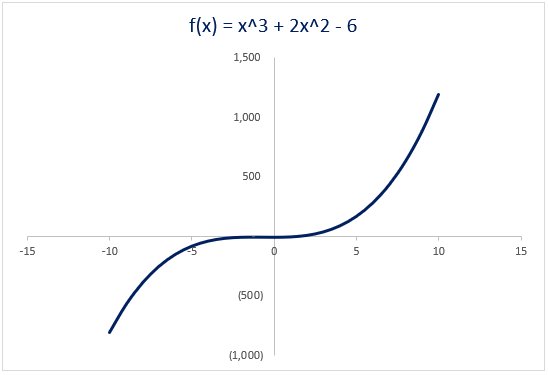We often use functions in finance. Most simple equations of the type y = f(x) we can plot in Excel (see attached figure). And such visual representation usually helps with analysis.

We model financial variables such as returns and prices via some probability distribution. We can plot the probability functions of the metric being a specific value or within a set range. These visual representations have different ‘shapes,’ as the underlying chances are differently distributed.

There is no limit to the ‘shape’ of a probability distribution. However, more complex ones may not have an easy equation to define them. If the distribution has a function that describes it, it is ‘closed-form,’ and we can use this function to calculate probabilities, confidence intervals, and run simulations like the Monte Carlo model. For most of the financial metrics, we do not know (and may never do) what the actual distribution is. We use historical data and estimates of the future. Therefore, the distributions we use in our models are mostly assumptions.

As you may have guessed already, we generally use closed-form ones in finance. There is an equation to use, and it has defined parameters. The main challenge we face is estimating these parameters accurately, as they will influence the ‘shape’ of the distribution.

## Types of Probability Distributions

In the most general terms, probability distributions can be either discrete or continuous.

In discrete distributions, a variable can only have specific predefined values. An example is a coin that can only be heads or tails. A die is another example; it can take any integer between 1 and 6 but cannot end up at 4.8.

In continuous distributions, on the other hand, variables can have any value, with some distributions having range limitations. In finance, we also face some monetary convention limits. As an example, a share price can be € 10.35 or € 10.36, but never € 10.355. Also, it can’t go below zero. These limits bring the share price values closer to having a discrete distribution, but in finance, we still consider such variables to be continuous. Examples are prices, return rates, interest rates, exchange rates, and others.

We present discrete distributions via histograms (bar charts) and continuous ones as curves. Sometimes, to support our modeling, we can look at continuous distributions as a discrete function with the number of discrete values approaching infinity.

## Probability Distributions

We will look at some of the most used distributions in financial modeling and financial analysis.

You can download the Excel model below the article to follow along in the overview of these probability distributions.

### Binomial Distribution

We use this to model events with binary outcomes. An example is the toss of a coin or an operation that can be either a success or a failure. Each occurrence is independent of the previous. If we have n instances, there are n+1 possible outcomes and 2^n possible paths to these outcomes. Binomial distributions are used a lot in valuing path-dependent options.

We can represent the probability of achieving x successes (tossing heads x times) with the following daunting formula: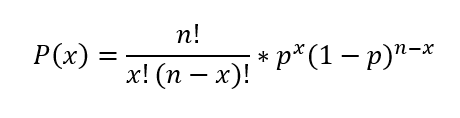Where the parameters are:

• p – probability for each trial;
• x – number of successes;
• n – number of tests.

Thankfully, we do not need to calculate this function, as Excel has it integrated – BINOM.DIST.

The expected value for a binomial distribution (or the mean) is equal to the number of trials multiplied by the probability for each trial: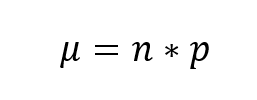We calculate the variance as follows: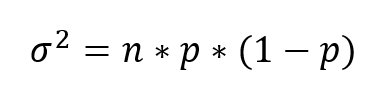The standard deviation of the distribution is the square root of the variance:If we want to calculate a random value within a binomial distribution for our financial model, we can use the following formula:### Uniform Distribution

Whenever we are modeling a variable where all possibilities are equally likely to occur, we have uniform probabilities. Without even knowing it, this is probably the one we use most in modeling and analysis. Every time you use the RAND or RANDBETWEEN function in Excel, you are applying a uniform probability distribution to the variable you are calculating.

There are two parameters to estimate for this distribution:

• a – lower bound;
• b – upper bound.

Then we can easily calculate the probability of an outcome with the simple formula: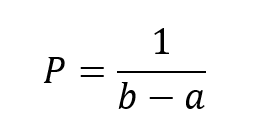The most expected value, or the mean, is calculated with the following formula:And the variance is as follows:A uniform distribution is one where all values have the same chance of being selected, so if we plot the probability distribution function for uniform distribution with lower bound of 5 and upper bound of 26, we get the following chart:For our financial models, we can calculate a random number within a uniform distribution by this formula:### Poisson Distribution

In this discrete distribution, random values can only be positive integers. Analysts use it to model the probability of an event occurring n times within a time interval when the average is known. As an example, we know that on a specific beach, five baby turtles are born each month. We can use a Poisson distribution to calculate the probability of having six turtles born in a single week.

There is only one parameter λ, which is both the mean and the variance. Knowing it, we can calculate the probability of x successes with the formula:Or we can use the Excel function, POISSON.DIST.

This distribution is useful for modeling operational risk, e.g., the number of defaults in a day, as part of credit risk analysis. The advantage it has over other probability distributions it that it only takes one parameter, which is a positive number.

On the example above, here is the calculation:In another example, say we have an average of seven red-light crossings per day at a given intersection. If we calculate the Probability Distributions for instances of 0 to 50 red-light crossings in a day, we can use the intervals between the cumulative probabilities and Excel’s VLOOKUP function to generate a random number for our model:And if we plot it on a chart, we get a sense of how likely instances are to occur.### Normal Distribution

The most common probability distribution is the normal distribution. It is continuous and has a distinct form when plotted, which is why we also refer to it as a bell curve.  One of the most common assumptions in financial modeling is that returns have a normal distribution.

The normal distribution has no skew and is perfectly symmetrical. We define the ‘standard’ normal distribution as one with a mean of zero and a standard deviation (and variance) of one. Approximately 68% of values fall within an interval of +/- one standard deviation from the mean. Then, around 95% fall within two standard deviations, and 99.7% – within three.

This one also has a daunting probability distribution function, but we can easily calculate it in Excel, using the NORM.DIST and NORM.INV functions.

In financial modeling, we mostly use this probability distribution for changes in quantity metrics and asset returns with constant volatility. An interesting fact is that the errors in real phenomena are often normally distributed.

If we have average monthly sales (our mean) of 25,400 and variance of 24,400, we can calculate random sales values within a normal distribution.The probability distributions when plotted look like this:### Lognormal Distribution

Analysts mostly use this probability distribution to model asset and equity prices under the assumption that their returns follow a normal distribution. We use average returns to model the prices, given some volatility. It also takes two parameters, a mean and variance. We will not look into formulas for those, as they are too complex to calculate by hand. Excel has a function that we can use instead – LOGNORM.DIST.

The standard lognormal distribution with a mean of zero and a standard deviation of one has the following curve when plotted.Using the same average sales of 25,400 and the standard deviation of 156.20, we can calculate random numbers to use in our financial models. However, we need to scale our mean and standard deviation and use the sized measures:### Beta Distribution

This distribution is useful in simulations of rates of recovery, as part of risk assessment. In Excel, we can calculate the Distribution Functions (PDF and CDF) with the BETA.DIST function.

Beta distributions have the following probability curve: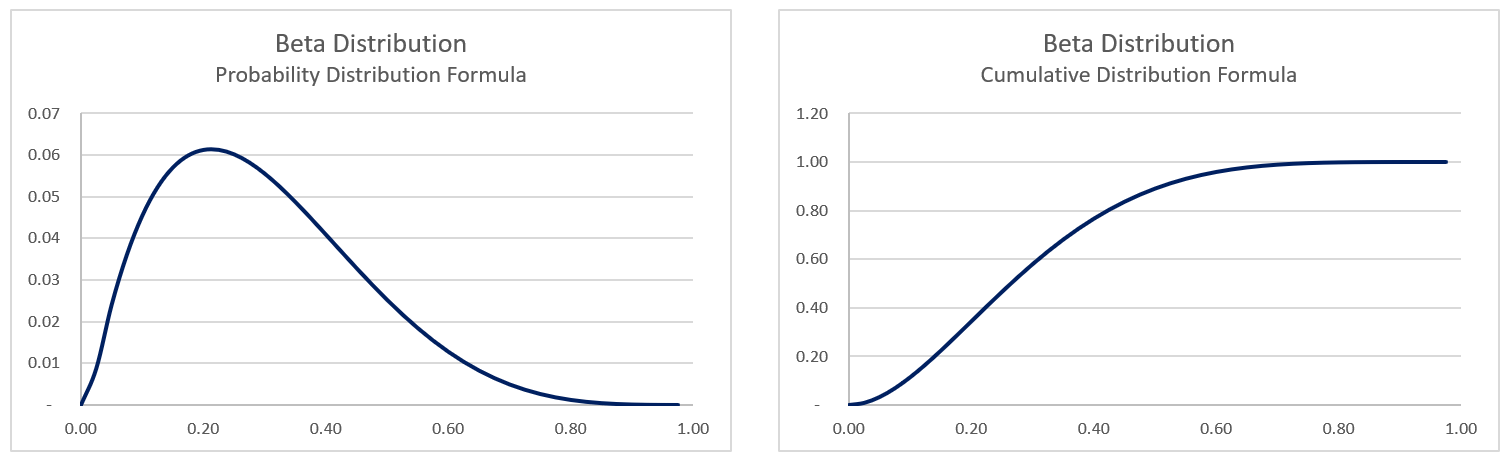To calculate random values for a variable with beta distribution, we use Excel’s BETA.INV formula like that: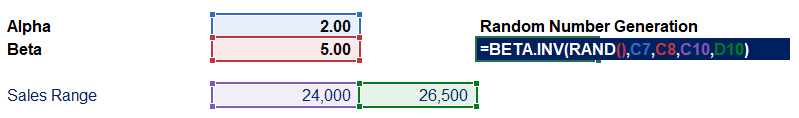### Student’s T DistributionWhenever we have samples of less than 30 instances from a population that we consider follows a normal distribution, we mostly use this. It has fatter tails on its plot, compared to the normal distribution. This results in higher value-at-risk for high confidence levels. Because of this, some risk managers prefer this distribution when modeling asset returns. The Excel function is T.DIST and takes only a single parameter – degrees of freedom, which is the sample size reduced by one (n – 1).

### Bivariate Distribution

So far, we looked at functions of the type y = f (x). The bivariate distribution helps us model functions with two variables, of the type z = f (x, y). Therefore the graphical representation is a 3-dimensional chart, with the x and y-axis showing the two variables and the z-axis – the probability. A normally distributed bivariate has a bell shape like the normal distribution. Risk analysts use this distribution in credit risk estimations. It is part of the CreditMetrics methodology by J.P. Morgan.

The Bivariate Normal Distribution looks like this when plotted in Excel: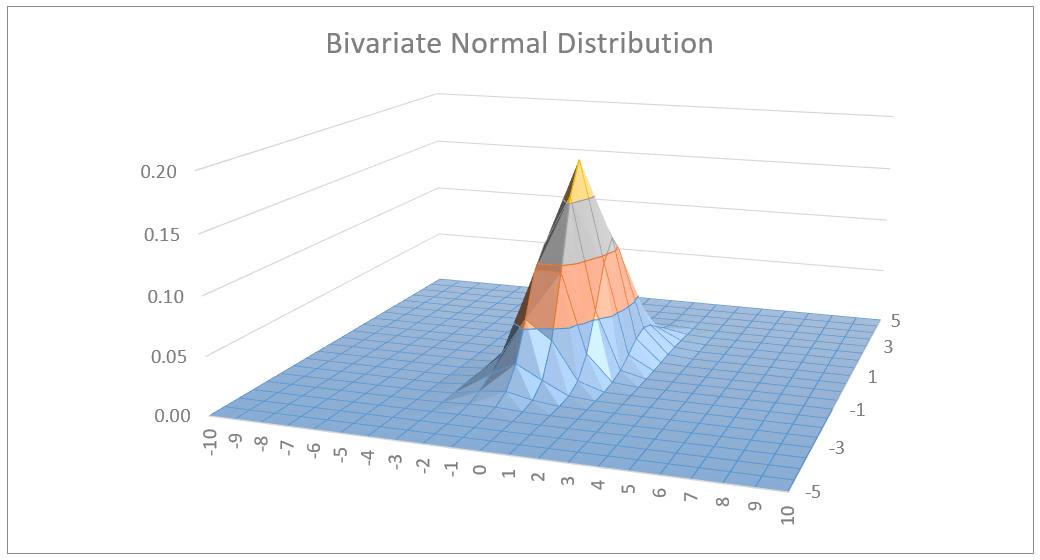## Conclusion

Financial modeling is always full of uncertainty. Even if we build the best model in technical terms, it will not serve its purpose if the underlying assumptions are incorrect. Therefore, it is essential to gain a proper understanding of the variables we are modeling and how best to represent their randomness. Using probability distributions can significantly improve our models and the usability of the results and findings we draw from them.

These were some of the most common distributions financial analysts use in their models to mitigate the effect of uncertainty and chance. This, in turn, improves the decision-making process in the business.

You can read our article on running Monte Carlo Simulations in Excel. It will further clarify the concepts outlined above, as it shows how we use some of these distributions in practice, to arrive at a more sophisticated model.

If you want to look through all the examples above, download our Excel model below.

## And Get a FREE Benchmark Analysis Template## FCCA, FMVA

Hi! I am a finance professional with 10+ years of experience in audit, controlling, reporting, financial analysis and modeling. I am excited to delve deep into specifics of various industries, where I can identify the best solutions for clients I work with.

In my spare time, I am into skiing, hiking and running. I am also active on Instagram and YouTube, where I try different ways to express my creative side.